# Catch up on the latest AI articles# ATC: Separating Unsupervised Learning From Reinforcement Learning!

3 main points
✔️ A proposal for a method of representation learning that can be decoupled from RL Agent learning
✔️ A new unsupervised learning task Augmented Temporal Contrast is proposed
✔️ To achieve high performance with ATC pre-trained encoders for a variety of tasks

Decoupling Representation Learning from Reinforcement Learning
written by
(Submitted on 9 Mar 2020)

Subjects: Machine Learning (cs.LG); Artificial Intelligence (cs.AI); Computer Vision and Pattern Recognition (cs.CV); Machine Learning (stat.ML)
Paper
COMM Code

## Technique

In this chapter, we introduce the newly proposed Augmented Temporal Contrast (ATC), which has the following structure: the input pair of observations $o_{t}$ and the pair of observations $o_{t+k}$ in the near future We then apply a stochastic data augmentation to observations for each training batch. We then apply a stochastic data augmentation to observations for each training batch. This paper uses random shift, which is very simple and easy to implement. The augmented observations are then moved into a small latent space by the encoder, and a contrastive loss is used to compute the loss for the feature. The goal of this task is for the encoder to extract meaningful information about the structure of the MDP from the observations.

This ATC can be divided into four components, as represented by the figure below: the first is a convolutional encoder $f_{\theta}$ with observation $o_{t}$ as input and the latent feature $z_{t} = f_{\theta}(\text{AUG}(o_{t}))$. The second one outputs a small latent code vector called linear global compressor $g_{\phi}$, which is a small latent code vector. The third one outputs a residual predictor MLP $h_{\psi}$, which represents a forward model that performs an operation like $p_{t} = h_{\psi}(c_{t})+c_{t}$. And finally, the contrastive transformation matrix $W$. $o_{t+k}$ is expressed as a target code through a process $\bar{c}_{t+k}=g_{\bar{\phi}}\left(f_{\bar{\theta}}\left(\operatorname{AUG}\left(o_{t+k}\right)\right)\right.$. The parameters processing this observation $o_{t+k}$, $\bar{\theta}$ and $\bar{\psi}$, are calculated by moving average of $\theta$ and $\psi$ which are the learned parameters and called momentum encoders.

$$\bar{\theta} \leftarrow(1-\tau) \bar{\theta}+\tau \theta ; \quad \bar{\phi} \leftarrow(1-\tau) \bar{\phi}+\tau \phi$$

Then, after using this task to pre-train encoders, only $f_{\theta}$ is used in the RL agent. The loss function for this task can be expressed using InfoNCE as follows

$$\mathcal{L}^{A T C}=-\mathbb{E}_{\mathcal{O}}\left[\log \frac{\exp l_{i, i+}}{\sum_{o_{j} \in \mathcal{O}} \exp l_{i, j+}}\right]$$

where $l$ is expressed as $l=p_{t} W \bar{c}_{t+k}$.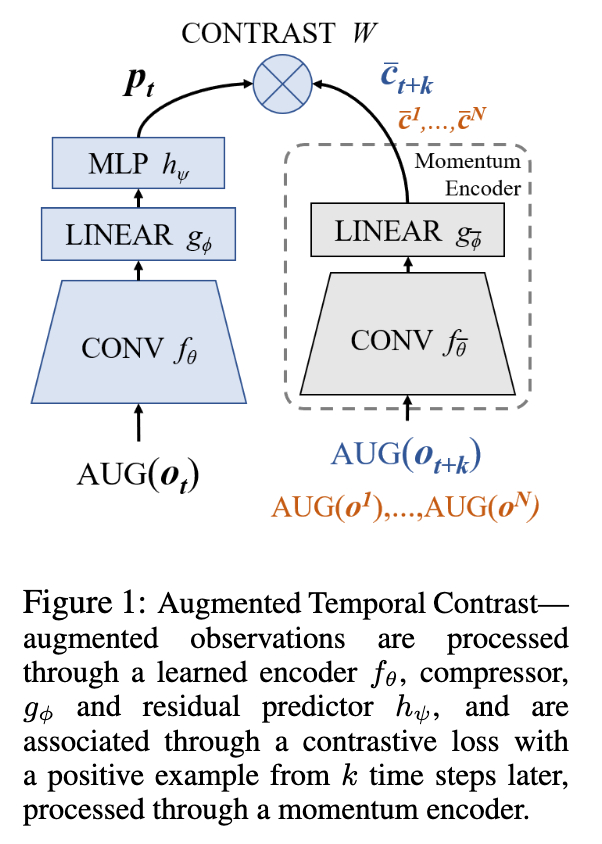## Experiment

### Environment and algorithms used for evaluation

In this paper, we evaluated ATC in three different environments: the DeepMind control suite (DMControl), Atari games, and the DeepMind Lab, where Atari uses discrete control (discrete actions such as right and left) In the game, DMLControl is a game that uses continuous control. And DMLab, DMLab is a task in which RL Agent acts in a visually complex 3D maze environment.

For the algorithms, we used and evaluated ATC for both on-policy and off-policy RL algorithms, and for DMMontrol, we used a random shift augmentation called RAD-SAC for soft actor We used a critic-based algorithm; for Atari and DMLab, we used PPO to evaluate it.

### Online RL and Unsupervised Learning

DMControl: Throughout this experiment, if the encoder is separated from the RL agent and the encoder learns online about the ATC task while at the same time the RL agent receives a presentation from the encoder and learns, the end-to-end RL case ( The following results show that the performance is equal or better than that of the traditional method (i.e., learning without ATC).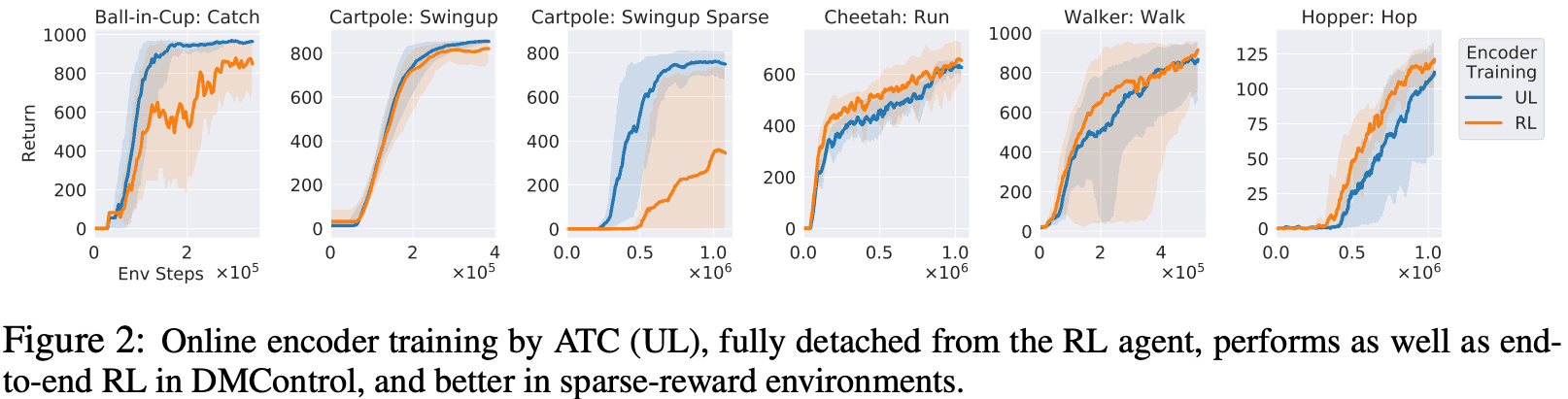DMLab: In our experiments with DMLab, we used two tasks: EXPLORE_GOAL_LOCATIONS, which involves moving around in a maze to find the goal, and LASERTAG_THREE_OPPONENTS, which involves quickly chasing enemies and tagging them. We experimented with two levels of two tasks of different magnitudes, for a total of four tasks: in the task on EXPLORE, the goal was found less frequently and the learning was less advanced, so, in our experiments, we use the method of prioritizing the values of $p \propto 1+R_{abs}$ (where $R_{a b s}=\sum_{t=0}^{n} \gamma^{t} \mid r_{t}$) and sampling the ones with higher priority for learning. If we designate this method as prioritized-UL (orange line below), it performs as well or better than the conventional RL (red line below), and it is an auxiliary task, Unsupervised The accuracy is about the same compared to using Learning, i.e., separating the encoder from the RL agent and not training it (green line).

Also for LASERTAG, the learning agent can see enemies well, but tagging them against enemies is a hard event to happen. In the case of traditional RL (red line on the right of the figure below), performance is very poor, especially for large labyrinths, and ATC is used to separate the agent from the RL agent. We also found that by doubling the frequency of updating the encoder, the performance was similar when compared to training without separating the encoder from the RL agent.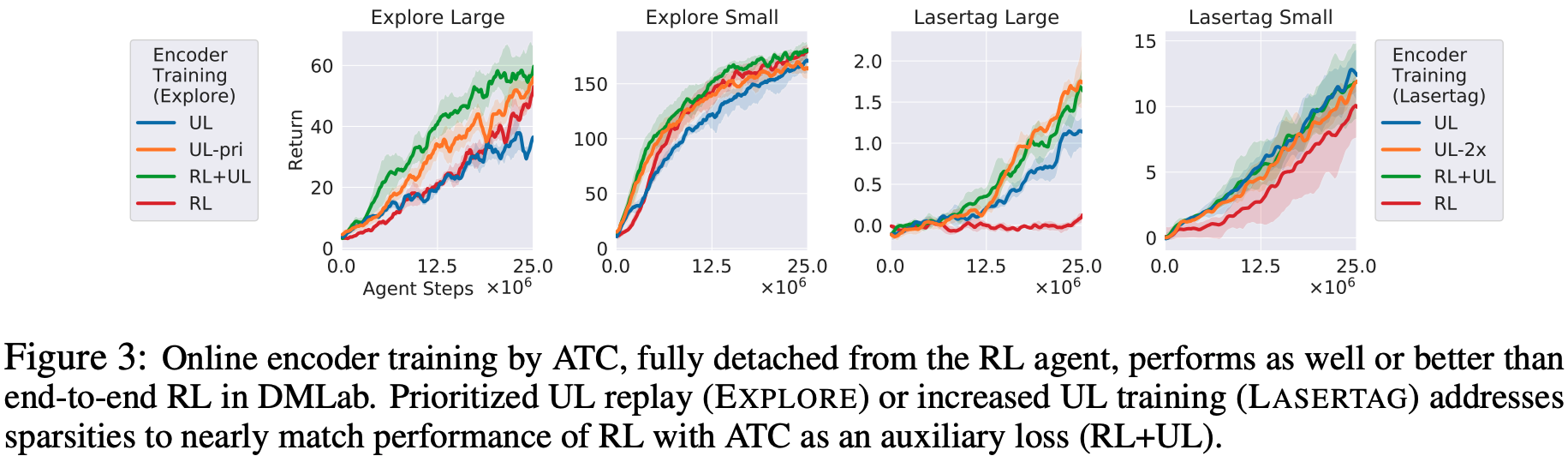Atari: In our experiments with Atari, we evaluated eight games and found that five of them performed better than end-to-end RL when the Encoder was decoupled from the RL Agent and trained using the ATC task. However, the performance of BREAKOUT and SPACE INVADERS was not as good as that of the RL Agent. However, when ATC was trained as an auxiliary task without separating it from the RL Agent, the performance of these and other tasks was better. In addition, when ATC was used to train the encoder for the first 100k transitions and to initialize the weight, the results showed that ATC was effective in some games.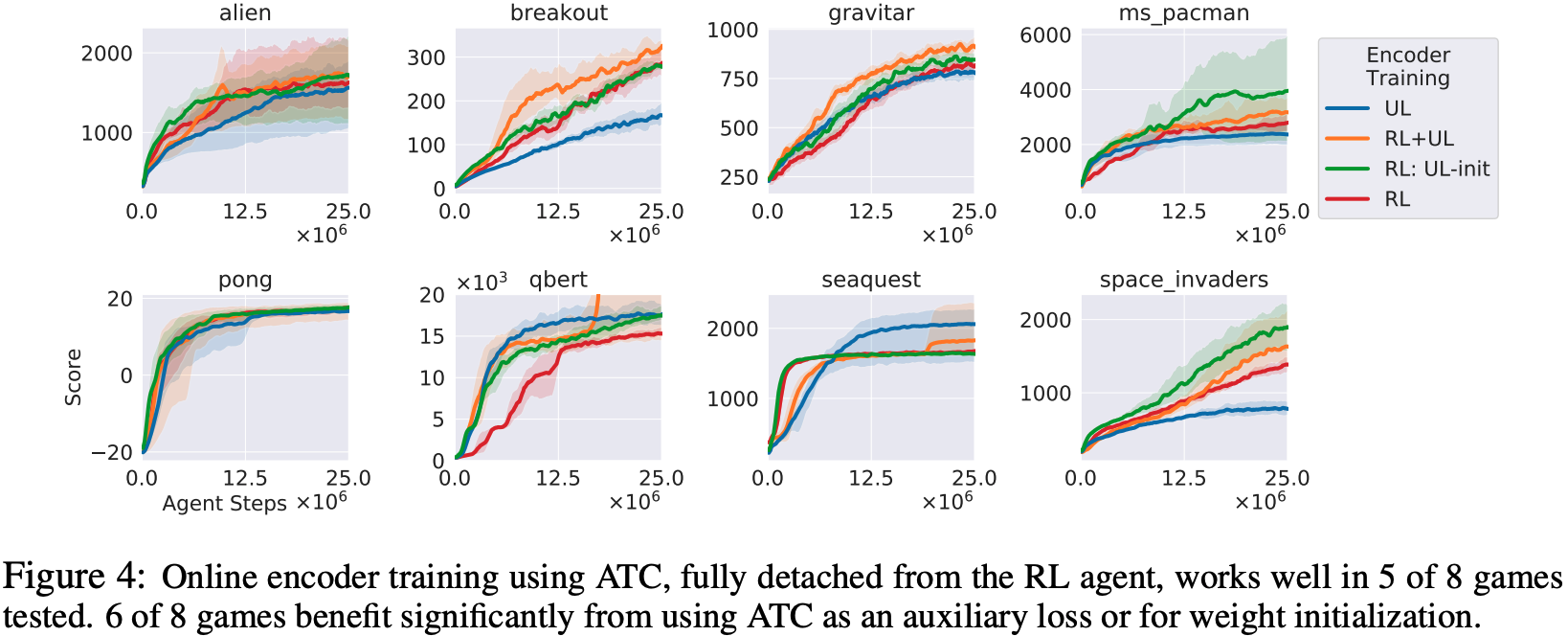### Benchmarking for Encoder pre-training

In this paper, we propose a new evaluation method as an evaluation against different unsupervised learning algorithms for RL. This evaluation is carried out in the following procedure.

1. Collect expert demonstrations from each environment
2. Using the data collected from the CNN encoder, we use unsupervised learning for pre-training.
3. We train the RL agent with a fixed weight of the encoder and evaluate its performance.

Here are the results for each environment.

DMControl: In this experiment, we used Augmented Contrast (AC), which is mainly used in CURL, and VAE as an unsupervised learning algorithm compared to ATC. As shown in the figure below, the performance of ATC is basically the same or better than other unsupervised algorithms. Also, ATC shows equal or better performance compared to end-to-end RL for all tasks.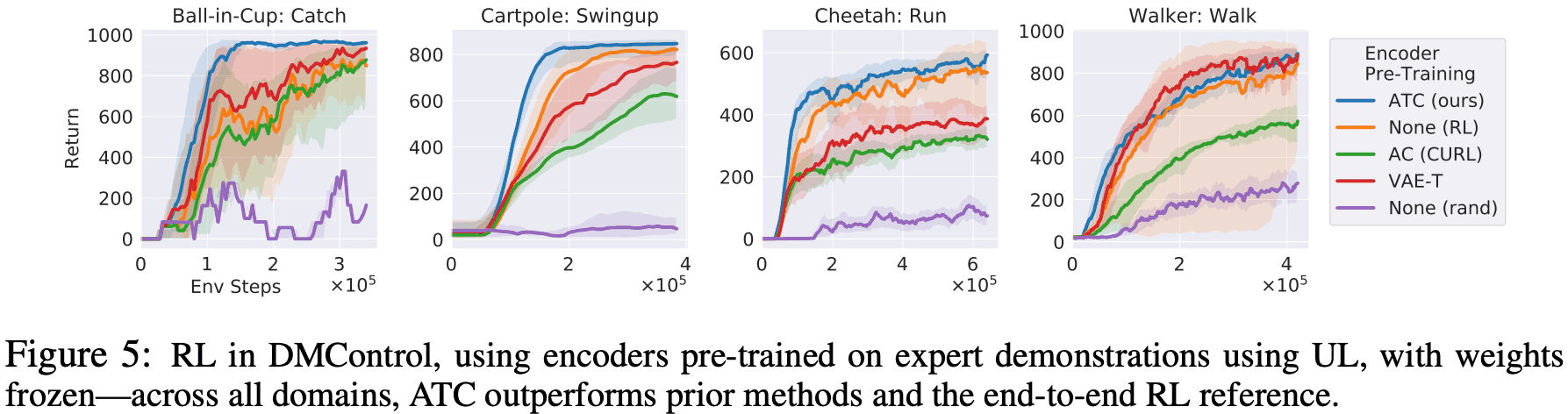DMLab: The following figure shows the results for DMLab. For the EXPLORE task, the performance is similar for all the tasks, but for Lasertag, the ATC one is the best The results can be seen in the figure below.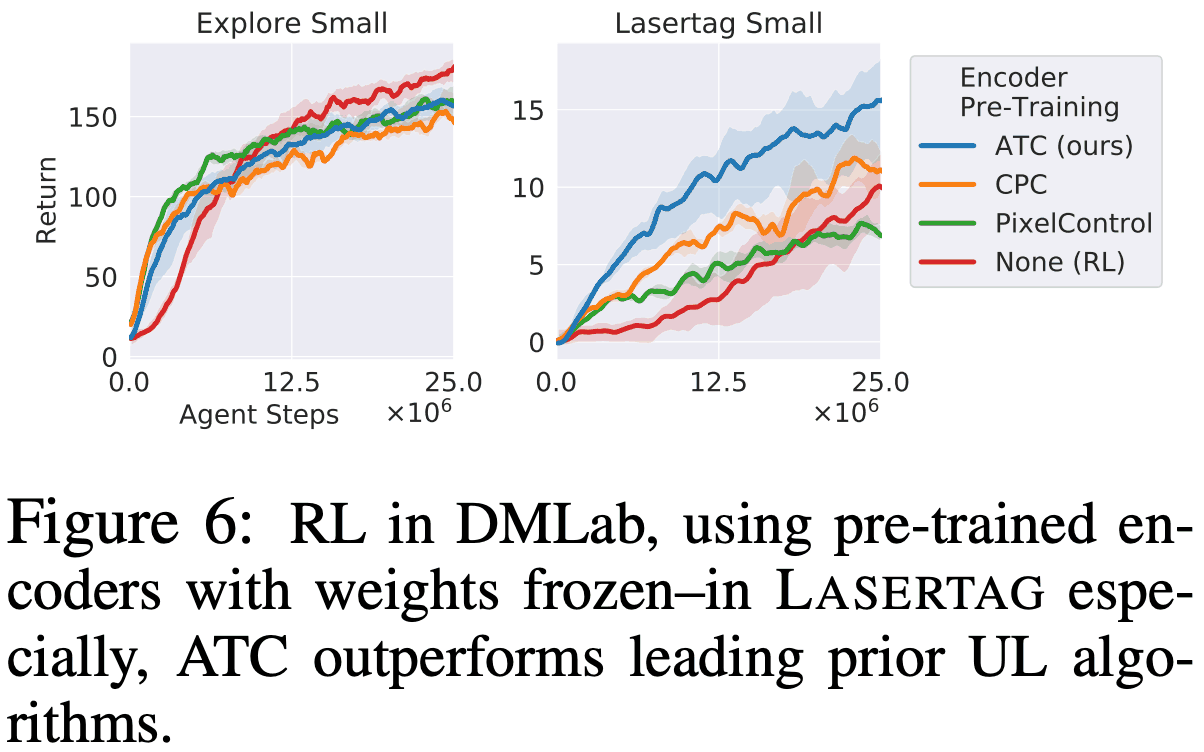Atari: For Atari, for the four games, ATC is trained by Pixel Control, VAE-T, and the Inverse model (which predicts the actions that transition to the pair of observations given two consecutive observations). model) and compared it to a method called Spatio-Temporal Deep InfoMax (ST-DIM). The results show that only ATC represents a higher performance than end-to-end RL in all three games, as the figure below shows.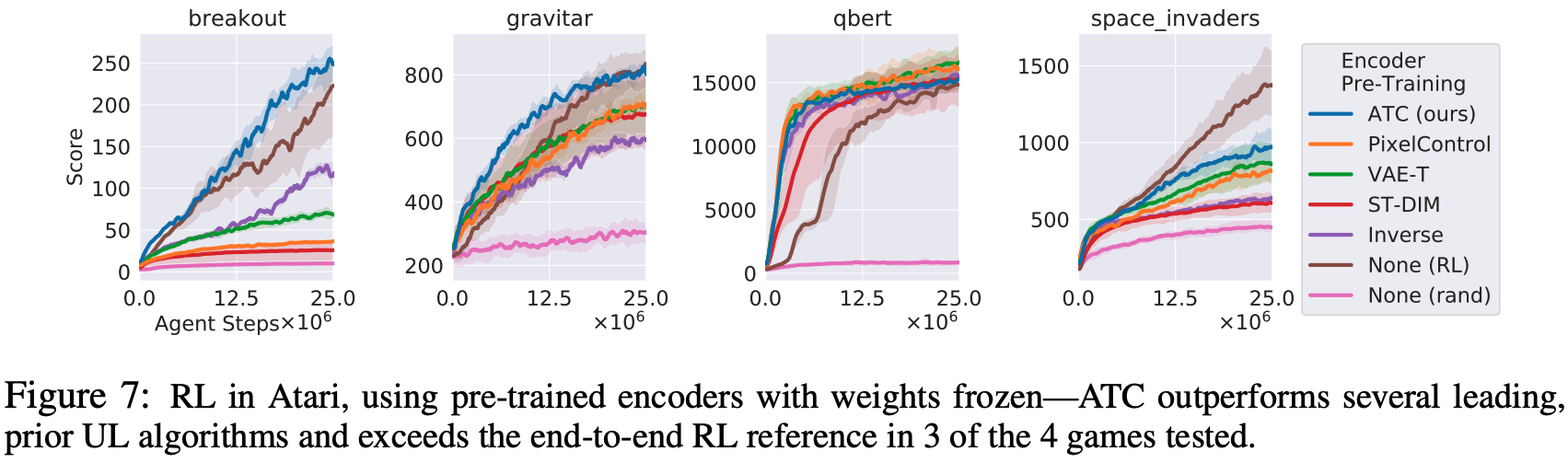This experiment was designed to evaluate whether ATC can train multi-task encoders by pre-training encoders using expert demonstrations on several environments and fixing the weight of the encoder and then using RL It trains an agent on another task and evaluates its performance.

DMControl: In this experiment, encoders are pre-trained by demonstrations of the Ball-in-Cup: Catch, Cartpole: Swingup, Cheetah: Run, Walker: Walk tasks in the top row below. The following figure shows the results of training an RL agent for each of the tasks in the bottom row of the figure, with the weight of the trained encoders fixed. The results in the bottom row show that the RL agent can be learned more efficiently by pre-training encoders using a different task and using the learned encoders compared to RL without pre-training, as shown in the bottom row below.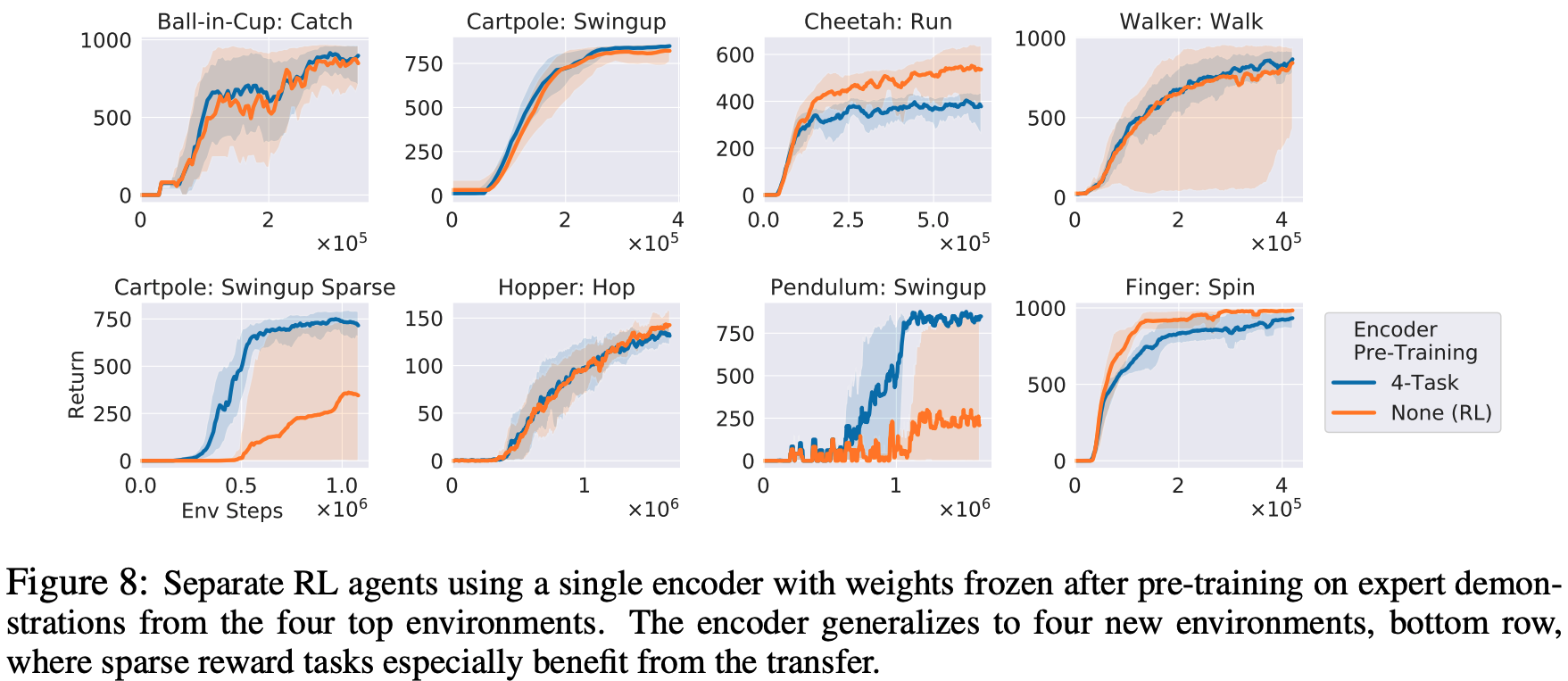### Ablation study and analysis of Encoder

About Random Shift: The experiments showed that the random shift image augmentation is effective for basically all environments. In the DMRControl experiment, we found that using a random shift during the training of the RL agent after fixing the encoder improves performance, rather than using a random shift only for training the encoder. This shows that augmentation constrains not only the representation of the encoder's output but also its policy and that during the training of the RL agent, rather than storing the images in a replay buffer, the encoder It would be faster and more efficient if we could store the 50-dimensional latent image vector through the replay buffer and use it for training. However, using the smallest possible random shift for this latent image has been ineffective because the shift is too large. In this paper, we propose a new method for data augmentation called subpixel random shift, which is based on linear interpolation between neighboring pixels.

An analysis of the use of adjacent transitions: Atari's For the Breakout task only, we found out that it is more effective to create and learn a match using a segment of the trajectory instead of creating a match based on individual transitions. The figure below shows a comparison between the case of using contrastive loss and similarity loss, where $T$ represents the time step ahead of the negative sample. As we can see from the figure below, the best performance is found for $T=16$. However, for other tasks, we have found that it performs well even when using individual transitions.

For trained Encoders: The right-hand side of the figure below shows a comparison between RL with and without random shift for unsupervised learning. From these results, we can see that unsupervised learning with RL agent and random shift focuses on the vicinity of the ball, whereas unsupervised learning without random shift mainly focuses on the paddle. You can see that they are paying attention to.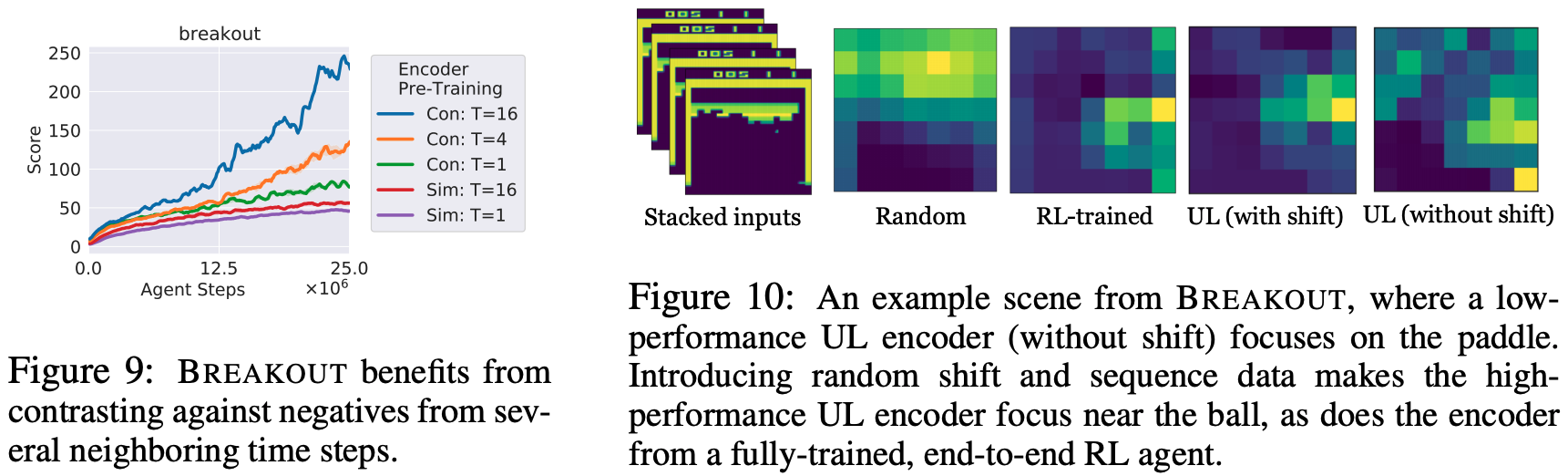## Summary

In this article, we have introduced an unsupervised learning task called ATC, which shows similar or improved performance compared to end-to-end RL performance even when the encoder is decoupled from the RL agent and pre-trained. The paper suggests that further analysis is necessary after conducting experiments in a variety of environments because there are some environments where the effect is not so pronounced, although the results are good in some environments. However, we believe that we will be able to learn the RL agent more flexibly and efficiently if it becomes possible to separate the encoder from the RL agent and learn it beforehand, so we would like to look forward to the future research.

If you have any suggestions for improvement of the content of the article,